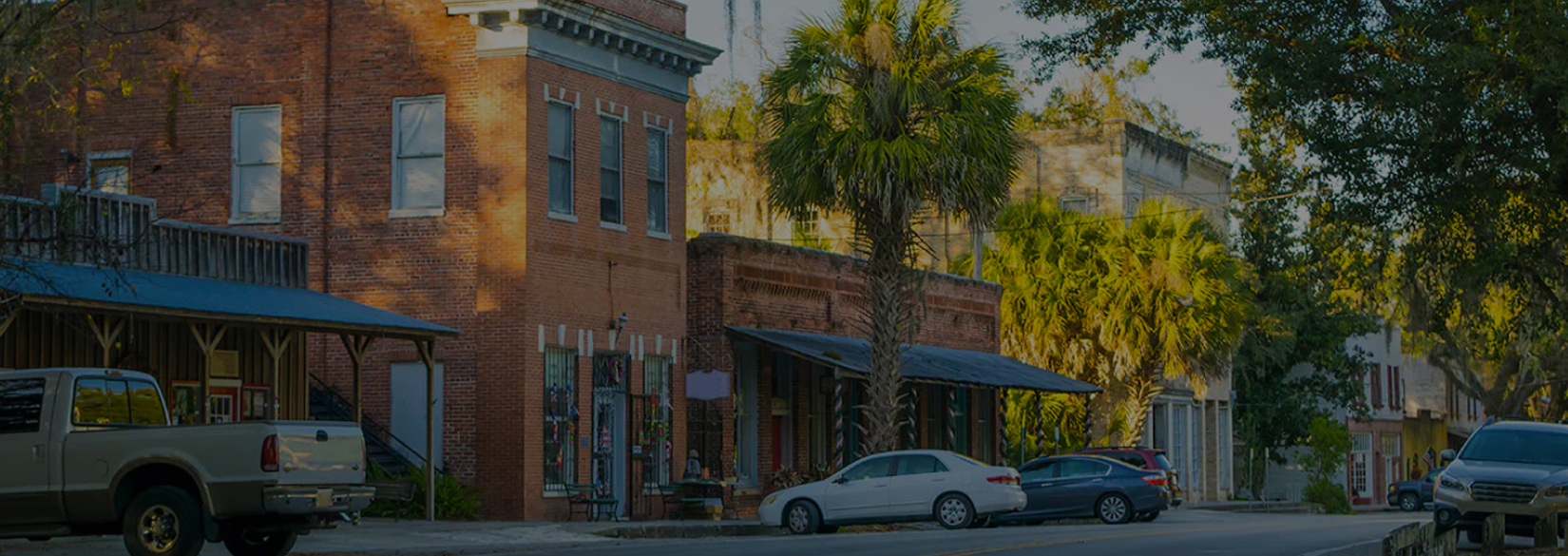# Case Study

## Remington RanchRemington Ranch is a 510 acre master planned community consisting of over 2000 homes located north of downtown Houston.## Problem

Here is where you add the problems. Here is where you add the problems. Here is where you add the problems. Here is where you add the problems. Here is where you add the problems. Here is where you add the problems. Here is where you add the problems.## Solution

Here is where you add the problems. Here is where you add the problems. Here is where you add the problems. Here is where you add the problems. Here is where you add the problems. Here is where you add the problems. Here is where you add the problems.## Outcome

Here is where you add the problems. Here is where you add the problems. Here is where you add the problems. Here is where you add the problems. Here is where you add the problems. Here is where you add the problems. Here is where you add the problems.## Website Design and Development

You can add the contents for this project here.